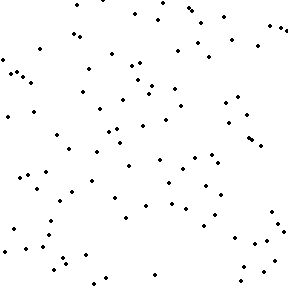# 图文讲解选择排序算法的原理及在python中的实现python代码实现：

def sort_choice(numbers, max_to_min=true):
“””

1、获取最大值x，将x移动到列最后。[n1, n2, n3, … nn]
2、将x追加到排序结果[n1, n3, … nn, n2]
3、获取排序后n-1个元素[n1, n3, … nn]，重复第一步，重复n-1次。
max_to_min是指从大到小排序，默认为true；否则从小到大排序。

sorted_numbers = []
[8, 4, 1, 0, 9], sorted_numbers = 
[4, 1, 0, 8], sorted_numbers = [9, 8]
[1, 0, 4], sorted_numbers = [9, 8, 4]
[0, 1], sorted_numbers = [9, 8, 4, 1]
, sorted_numbers = [9, 8, 4, 1, 0]
“””
if len(numbers) numbers[max_index]:
max_index = i
else:
if num < numbers[max_index]: max_index = i numbers = numbers[:max_index] + numbers[max_index + 1:] + [numbers[max_index]] return numbers

>>> get_left_numbers([0, 4, 0, 31, 9, 19, 89,67], get_max=true)
[0, 4, 0, 31, 9, 19, 67, 89]
>>> get_left_numbers([0, 4, 0, 31, 9, 19, 89,67], get_max=false)
[4, 0, 31, 9, 19, 89, 67, 0]
>>> sort_choice([0, 4, 0, 31, 9, 19, 89,67], max_to_min=false)
[0, 0, 4, 9, 19, 31, 67, 89]
>>> sort_choice([0, 4, 0, 31, 9, 19, 89,67], max_to_min=true)
[89, 67, 31, 19, 9, 4, 0, 0]

Posted in 未分类